# Finding Square Root Through Prime Factorisation

## Notes

### Finding Square Root Through Prime Factorisation:

Consider the prime factorisation of the following numbers and their squares.

 Prime factorisation of a Number Prime factorisation of its Square 6 = 2 × 3 36 = 2 × 2 × 3 × 3 8 = 2 × 2 × 2 64 = 2 × 2 × 2 × 2 × 2 × 2 12 = 2 × 2 × 3 144 = 2 × 2 × 2 × 2 × 3 × 3 15 = 3 × 5 225 = 3 × 3 × 5 × 5

You will find that each prime factor in the prime factorisation of the square of a number, occurs twice the number of times it occurs in the prime factorisation of the number itself.

1) Find the square root of 324?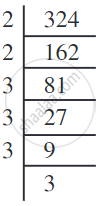By pairing the prime factors, we get
324 = 2 × 2 × 3 × 3 × 3 × 3
= 22 × 32 × 32
= (2 × 3 × 3)2

So, sqrt(324) = 2 × 3 × 3 = 18.

2) Find the square root of 256?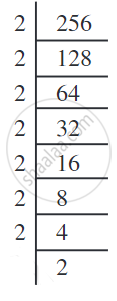Prime factorisation of 256 is
256 = 2 × 2 × 2 × 2 × 2 × 2 × 2 × 2
By pairing the prime factors we get,
256 = 2 × 2 × 2 × 2 × 2 × 2 × 2 × 2
= (2 × 2 × 2 × 2)2
Therefore,
sqrt(256) = 2 × 2 × 2 × 2 = 16

## Example

Find the square root of 6400.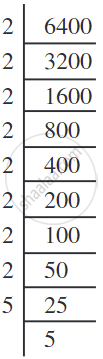6400 = 2 × 2 × 2 × 2 × 2 × 2 × 2 × 2 × 5 × 5
Therefore, sqrt(6400) = 2 × 2 × 2 × 2 × 5 = 80.

## Example

Is 90 a perfect square?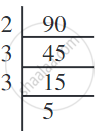90 = 2 × 3 × 3 × 5
The prime factors 2 and 5 do not occur in pairs.
Therefore, 90 is not a perfect square.
That 90 is not a perfect square can also be seen from the fact that it has only one zero.

## Example

Is 2352 a perfect square? If not, find the smallest multiple of 2352 which is a perfect square. Find the square root of the new number.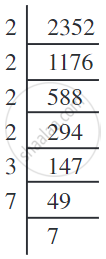We have 2352 = 2 × 2 × 2 × 2 × 3 × 7 × 7
As the prime factor 3 has no pair, 2352 is not a perfect square.
If 3 gets a pair then the number will become perfect square. So, we multiply 2352 by 3 to get,
2352 × 3 = 2 × 2 × 2 × 2 × 3 × 3 × 7 × 7
Now each prime factor is in a pair.
Therefore, 2352 × 3 = 7056 is a perfect square.
Thus the required smallest multiple of 2352 is 7056 which is a perfect square.
And, sqrt(7056) = 2 × 2 × 3 × 7 = 84.

## Example

Find the smallest square number which is divisible by each of the numbers 6, 9, and 15.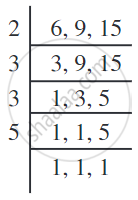The least number divisible by each one of 6, 9, and 15 is their LCM.
The LCM of 6, 9 and 15 is 2 × 3 × 3 × 5 = 90.
Prime factorisation of 90 is 90 = 2 × 3 × 3 × 5.
The prime factors 2 and 5 are not in pairs. Therefore 90 is not a perfect square.
In order to get a perfect square, each factor of 90 must be paired. So we need to
make pairs of 2 and 5. Therefore, 90 should be multiplied by 2 × 5, i.e., 10.
Hence, the required square number is 90 × 10 = 900.
If you would like to contribute notes or other learning material, please submit them using the button below.

### Shaalaa.com

Finding Square Roots through The Prime Factorisation [00:12:49]
S
0%